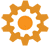Welcome to EnviroDIY, a community for do-it-yourself environmental science and monitoring. EnviroDIY is part of WikiWatershed, a web toolkit designed to help citizens, conservation practitioners, municipal decision-makers, researchers, educators, and students advance knowledge and stewardship of fresh water. New to EnviroDIY? Start here

# Email alerts rough draft. Free to use

Home Forums Miscellaneous Email alerts rough draft. Free to use

• Author
Posts
• #17963

Hey everyone! Here is a python program I wrote that downloads the csv file from monitor my water shed about a specific site and allows you to set up email alerts based on the readings. I will clean it up and put it on github eventually but if you want to check it out and play around it is posted below! Any suggestions or questions are welcome.

Requirements:

smtp server/account (elastic mail or sendgrid)

a computer that’s on 24/7 or a cloud computing account. (google cloud or amazon cloud computing)

I run this on a google cloud machine 24/7 but I think I’m going to switch to an amazon webserver soon as I believe they have a free option that will work.

############################################################################################################################
# #
# Email Alerts for Strawberry Creek restoration program. #
# This program downloads sensor csv data of mayfly’s located around UCB campus from envirodiy.com. #
# It allows you to program email alerts to a the list below if a threshold is met. #
# The threshold is measured in standard deviations either above or below the mean and can be changed #
# by adjusting the cushion values. The larger the cushion the more extreme of a deviation is needed to trigger an email. #
# The time_between_readings variable should be set to the mayfly it is monitoring. The cycles until next alert variables #
# Written by Andrew Glaros #
# Last edited by strawberry creek intern Andrew Glaros on 6/28/2023 #
# #
############################################################################################################################

from datetime import datetime, timedelta
import requests
import csv
import time
import io
import pandas as pd
import numpy as np
import smtplib
from email.message import EmailMessage

#below are variables that can be changed to coustomize this program.
site_name=’Grinell’
url = ‘url that links to csv file of site of interest’
#Location:Roach Menos EH&S

#smtp account info
username = ’email_associated with smtp account’
pswd = “password for smtp accound”
url_email= ‘url to be sent in the body of the email’
smtp_sever_url = “smtp.elasticemail.com”
smtp_port=2525
fromAddr = ’email associated with smtp accound’

#cushions are measured in standard deviations.
temp_trigger_cushion=2.8
cond_trigger_cushion=2.7
depth_trigger_cushion=2.8
#battery_trigger_cushion=2.8 not in use

#should be set to the same as the mayfly. measured in seconds

#hours to go back to find average and sd
hours=48

################################################################
# #
# Only edit under here if you want to change the code yourself #
# #
################################################################

from datetime import datetime, timedelta
import requests
import csv
import time
import io
import pandas as pd
import numpy as np
import smtplib
from email.message import EmailMessage

def threshold(array,name,cushion):
mean=np.mean(array)
std=np.std(array)
high_end=mean+cushion*std
low_end=mean-cushion*std
print(‘current ‘, name,’:’, array[-1], ’48 hour mean:’,np.round(mean,3), ‘ 48 hour std: ‘, np.round(std,3))
return [low_end, high_end]

def send_email(array, name):

msg = EmailMessage()
msg[‘Subject’] = subject
mean=np.round(np.mean(array),3)
std=np.round(np.std(array),3)
body = f’Email for {name} was called from the {site_name}.\nThe current reading is {current_reading}. The 48 hour mean is {mean}. The 48 hour sd is {std}\n\nSensor URL: {url_email}’
print(body)

msg.set_content(body)
smtp_sever = smtplib.SMTP(smtp_server_url, smtp_port)
server.send_message(msg)
server.quit()

def condition_tester(low_end,high_end,array,name):
if float(array[-1])<=low_end or float(array[-1])>=high_end:
send_email(array,name)
return True

while True:
voltage_count += 1
temp_count += 1
cond_count += 1
depth_count += 1

response = requests.get(url)

if response.status_code == 200:
csv_data = response.text

# Skip the header line and lines starting with a hash symbol
csv_data_lines = [line for line in csv_data.splitlines()[1:] if not line.startswith(“#”)]

# Create a StringIO object to simulate a file-like object
csv_file = io.StringIO(‘\n’.join(csv_data_lines))

try:
df[‘DateTime’] = pd.to_datetime(df[‘DateTime’]) # Convert to datetime data type

# Calculate the starting point for the last 48 hours and filter table
current_time = datetime.now()
start_time = current_time – timedelta(hours=hours)
last_48_hours_data = df[df[‘DateTime’] >= start_time]

# get the data in arrays
voltage_array=(last_48_hours_data[‘EnviroDIY_Mayfly_Batt’]).values
temp_array=(last_48_hours_data[‘Meter_Hydros21_Temp’]).values
cond_array=(last_48_hours_data[‘Meter_Hydros21_Cond’]).values
depth_array=(last_48_hours_data[‘Meter_Hydros21_Depth’]).values

except pd.errors.ParserError as e:
# Print the error and the problematic line
print(“ParserError:”, e)
print(“Problematic line:”, csv_data_lines)

# low_end_threshold_voltage , high_end_threshold_voltage = threshold(voltage_array,’voltage’,battery_trigger_cushion)
# reset=condition_tester(low_end_threshold_voltage,high_end_threshold_voltage,voltage_array, ‘voltage’)
# if reset==True:
# voltage_count=0

low_end_threshold_temp, high_end_threshold_temp = threshold(temp_array,’temp’,temp_trigger_cushion)
reset=condition_tester(low_end_threshold_temp,high_end_threshold_temp,temp_array, ‘temp’)
if reset==True:
temp_count=0

low_end_threshold_cond , high_end_threshold_cond = threshold(cond_array,’cond’,cond_trigger_cushion)
reset=condition_tester(low_end_threshold_cond,high_end_threshold_cond,cond_array, ‘cond’)
if reset==True:
cond_count=0